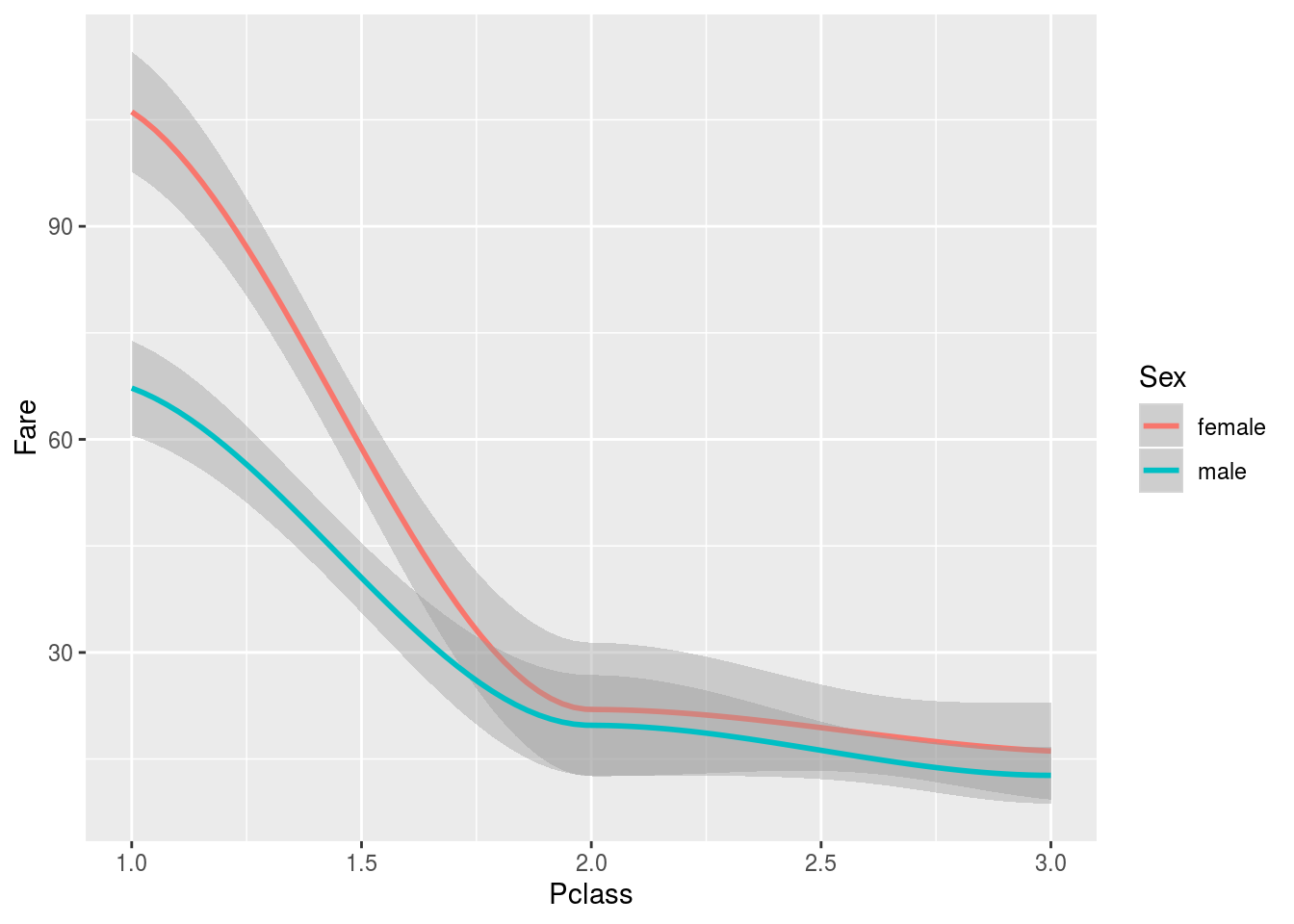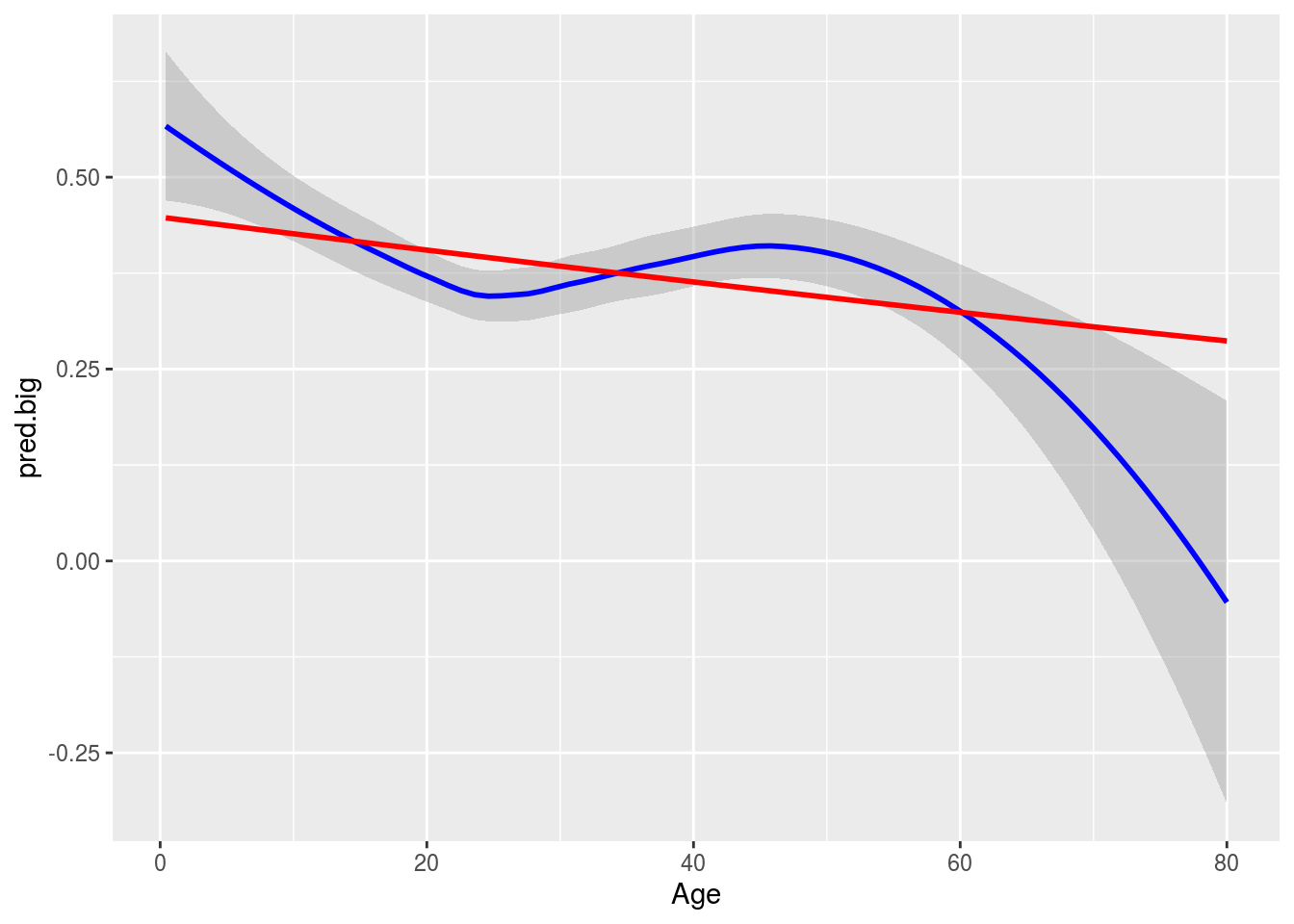## Problem 1: Extracting the Last Name with strsplit and sapply

### Problem 1(a)

Make a function that takes in a data frame in the same format as Titanic, and returns the percentage (i.e., a number between 0 and 100) of people who did not survive

#### Solution

precent.n.survived <- function(titanic){
100*(1-mean(titanic$Survived)) } titanic <- read.csv("http://guerzhoy.princeton.edu/201s20/titanic.csv") precent.n.survived(titanic) ##  61.44307 Learning goal: use the fact that the mean of a vector of logicals is the proportion of TRUEs there. (And functions.) ### Problem 1(b) You can use strsplit to split character strings into words. For example, the following splits a character string into words assuming that the words are separated by a space words <- strsplit("Go Tigers", " ")[] words ##  "Go" "Tigers" words ##  "Go" words ##  "Tigers" Write a function that takes in the name of a person (as it appears in the Titanic dataset) and returns the persons last name #### Solution get.last.name <- function(full.name){ words <- strsplit(as.character(full.name), " ")[] words[length(words)] } Learning goal: understand the specifications for a new function, and use that function to achieve a task ### Problem 1(c) Add a column to the titanic dataset that contains just the last name for each row. You should use sapply and your function from Problem 1(b) #### Solution titanic <- titanic %>% mutate(Last.Name = sapply(X = Name, FUN = get.last.name)) Learning goal: use sapply to compute a new column of a dataframe by applying a function to each element of an existing column. That is: combine using sapply to compute a function of each element of a vector with extracting and adding columns from/to data frames. ## Problem 2: Predicting using the last name ### Problem 2(a) Use logistic regression to predict survival using the last name of a person. Are you able to obtain a better accuracy than the baseline classifier? Compute and compare the false positive rate (FPR), false negative rate (FNR), and the positive predictive value (PPV). The definitions are as follows: $FPR = \frac{\text{# of times the model said "positive" and was wrong}}{\text{# of negatives }}$ $FNR = \frac{\text{# of times the model said "negative" and was wrong}}{\text{# of positives }}$ $PPV = \frac{\text{# of times the model said "positive" and was correct}}{\text{# of times the model said "positive"}}$ Can you come up with a theory that would explain why you can predict survival using the last name? #### Solution We’ll build the model using the entire dataset. fit <- glm(Survived ~ Last.Name, family = binomial, data = titanic) pred <- predict(fit, newdata = titanic, type = "response") > .5 mean(pred == titanic$Survived)   
##  0.9289741

Most of what’s going on is that the last name identifies just one person in the dataset, for whom the answer is known. So it’s not surprising that the predictions are good.

Learning goal: understand that when predicting using a categorical variable, we are predicting the average for each category, and apply this fact to a new situation. Be able to run logistic regression in R.

FPR <- sum(pred == T & titanic$Survived == 0)/sum(titanic$Survived==0)
FNR <- sum(pred == F & titanic$Survived == 1)/sum(titanic$Survived==1)
PPV <- sum(pred == T & titanic$Survived == 1)/sum(pred == T) Learning goal: operate on logical vectors with boolean operators. Translate the definitions of FPR/FNR/PPV/… into code. ### Problem 2(b) One idea is to try to predict using the last name, but for different people than the ones in the training set. names.test <- titanic %>% group_by(Last.Name) %>% mutate(num = n()) %>% filter(num >= 2) %>% summarize(Name = Name) %>% arrange(Last.Name) names.train <- titanic %>% group_by(Last.Name) %>% mutate(num = n()) %>% filter(num >= 2) %>% summarize(Name = Name) %>% arrange(Last.Name) test.set <- titanic %>% filter(Name %in% names.test$Name)
train.set <- titanic %>% filter(Name %in% names.train$Name) fit <- glm(Survived ~ Last.Name, family = binomial, data = train.set) ## Warning: glm.fit: algorithm did not converge mean((predict(fit, newdata = train.set, type = "response") > 0.5) == train.set$Survived)
##  1
mean((predict(fit, newdata = test.set, type = "response") > 0.5) == test.set$Survived) ##  0.6766917 Note that there actually is a correlation between the survival of people with the same name, since they are usually related. Learning goal: demonstrate an intuitive understanding of overfitting. ### Problem 3 ### Problem 3: ggplot Use ggplot to visualize the relationship between the fare and the class, as well as the sex of the passenger. Do you see any patterns? #### Solution ggplot(data = titanic, mapping = aes(x = Pclass, y = Fare)) + geom_smooth(mapping = aes(color = Sex), method = "loess")Learning goal: display the relationship between three variables on one plot using ggplot. We see that the average fare for male passengers was smaller than for female passengers, in first class. If you look further, you’ll find that the fare is actually recorded per family. ### Problem 4: ggplot Use ggplot to visualize the relationship between the age of a passenger and their probability of survival, based on a model the uses the passenger’s age, sex, class, and fare, as well as based on a model that uses just the passenger’s age. Superimpose the two plots. #### Solution fit.big <- glm(Survived ~ Age + Sex + Pclass, family = binomial, data = titanic) fit.small <- glm(Survived ~ Age, family = binomial, data = titanic) titanic[, "pred.big"] <- predict(fit.big, newdata = titanic, type = "response") titanic[, "pred.small"] <- predict(fit.small, newdata = titanic, type = "response") ggplot(data = titanic, mapping = aes(x = Age)) + geom_smooth(mapping = aes(y = pred.big), method = "loess", color = "blue") + geom_smooth(mapping = aes(y = pred.small), method = "loess", color = "red")Learning goal: use ggplot to display the predictions of a model; display several curves on one plot using ggplot. ### Problem 5: Insurance rates Write a function that will compute the total profit (or loss) if the if the insurance agent uses a logistic regression model that takes that uses the sex, class, and age of a person to predict whether they will survive, for the population of people on the titanic (note: this is “cheating,” since in reality an agent would not have access to the data to fit their model; there are also other issues here, which we will discuss). The policy the insurance agent uses the following procedure: • If the person’s probability of survival is less than p, turn them away. • If the person’s probability of survival is greater than or equal to p, sell them insurance for premium. • If the person does not end up surviving, pay benefit to the estate. Find reasonable p, premium, and benefit which would yield a profit in the case of the Titanic. You should do that by just trying to call your function manually using different values. (Again, note: this is an exercise, and not a realistic example. Most liners did not sink; no insurer would sell insurance if they knew the liner would sink. In fact, insurers often refuse to sell insurance when the probability of a bad outcome is not very small.) #### Solution • If the person’s probability of survival is less than p, turn them away. • If the person’s probability of survival is greater than or equal to p, sell them insurance for premium. • If the person does not end up surviving, pay benefit to the estate. total.profit <- function(fit, p, premium, benefit, dat){ dat[, "pred"] <- predict(fit, newdata=titanic, type = "response") n.accepted <- sum(dat$pred > p)
n.paid.benefit <- sum( (dat$pred > p) & (dat$Survived == 0) )

total.expediture <- n.paid.benefit * benefit

total.revenue - total.expediture
}

p <- 0.90
total.profit(fit, p, premium, benefit, titanic)
##  16800